Function Repository Resource:

# SubsetsWithComplements

Get a list of subsets of a list along with their complements

Contributed by: Seth J. Chandler
 ResourceFunction["SubsetsWithComplements"][list] gives a List of all possible subsets of list along with their complements. ResourceFunction["SubsetsWithComplements"][list,n] gives all subsets containing at most n elements along with their corresponding complements. ResourceFunction["SubsetsWithComplements"][list,{n}] gives all subsets containing exactly n elements. ResourceFunction["SubsetsWithComplements"][list,{nmin,nmax}] gives all subsets containing between nmin and nmax elements. ResourceFunction["SubsetsWithComplements"][list,nspec,s] limits the result to the first s subsets. ResourceFunction["SubsetsWithComplements"][list,nspec,{s}] gives, if possible, the sth subset and complement.

## Details and Options

The permissible argument specifications are exactly the same as for Subsets.

## Examples

### Basic Examples (2)

Get all the subsets of a List of characters, paired with their complements:

 In:=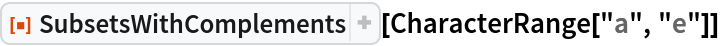Out=Take a List of three characters and get all the one-element subsets thereof and their complements:

 In:=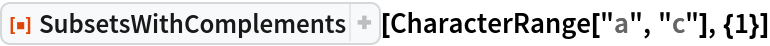Out=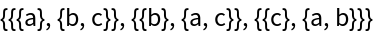### Scope (4)

Get all subsets from a List of five characters with at most two elements, along with their complements:

 In:=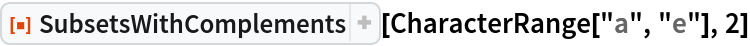Out=Take a List of five characters and get all subsets with between two and three elements and their complements:

 In:=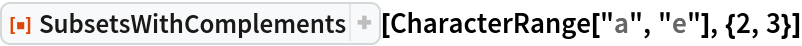Out=Get the first three two-element subsets and complements from a list with six elements:

 In:=Out=Get the seventh two-element subset and complements of a list with six elements:

 In:=Out=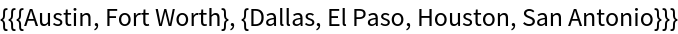### Applications (2)

Find and show conditions under which each expression in a List will have a value greater than that of any other expression in the list:

 In:=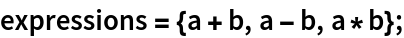In:=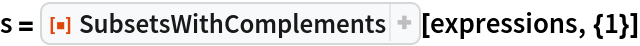Out=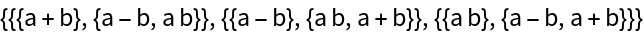In:=In:=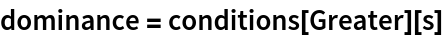Out=In:=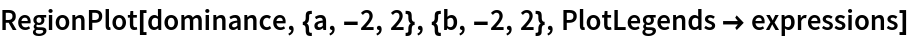Out=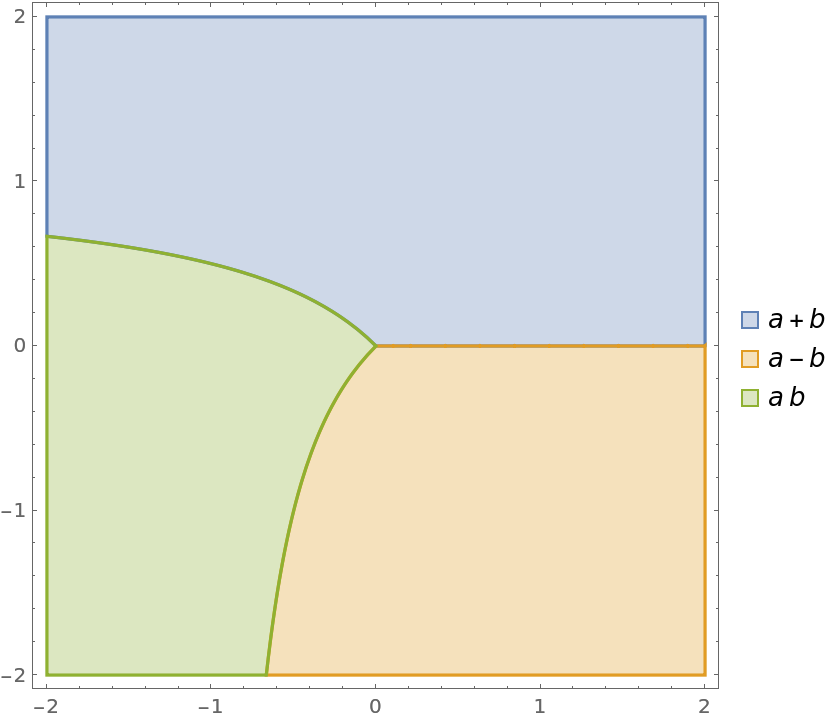Do similarly for the lowest value of any expression in the List:

 In:=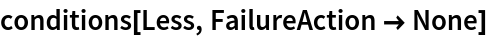In:=Out=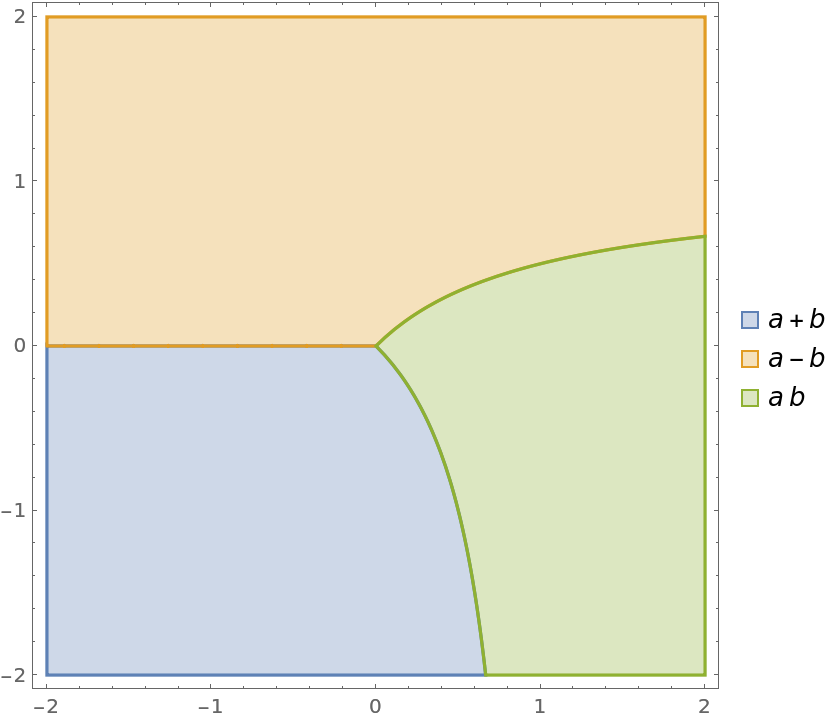### Neat Examples (1)

A certain classifier predicts the probabilities that a person will be in each of three possible classes. A misclassification results in a utility of –1, except when a person is really in the first class but is misclassified as being a member of the second class. This latter case results in a utility of –u, where u is some non-negative number. For each value of u, find and show the set of classifier probabilities {p1,(1–p1)p2,1–(p1+(1–p1)p2)} that result in each prediction being the one that maximizes expected utility:

 In:=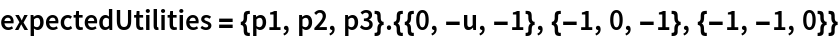Out=In:=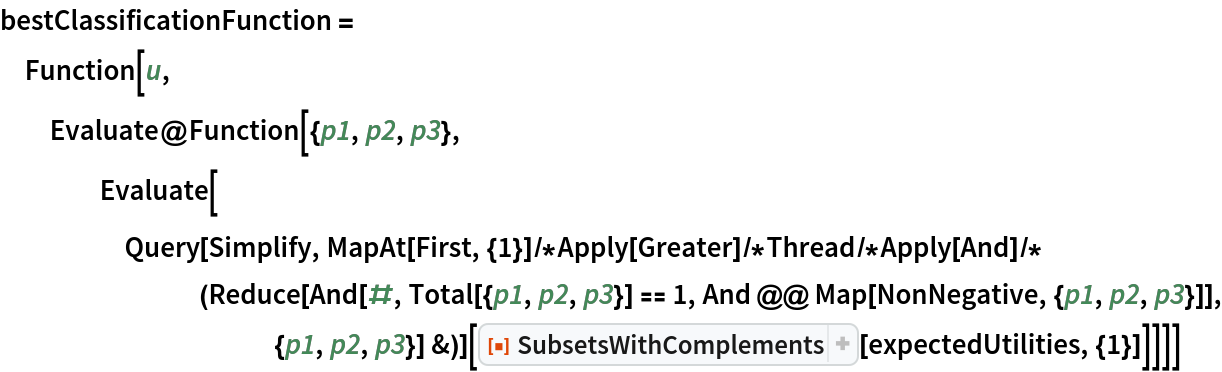Out=In:=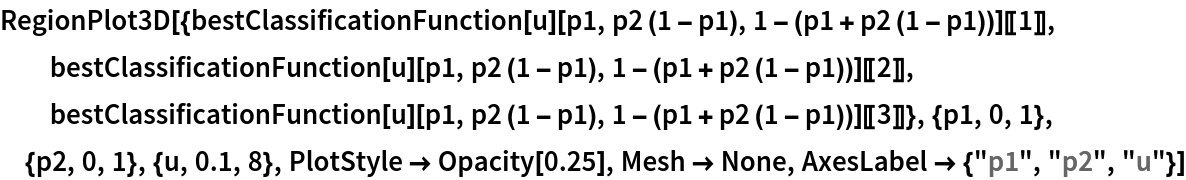Out=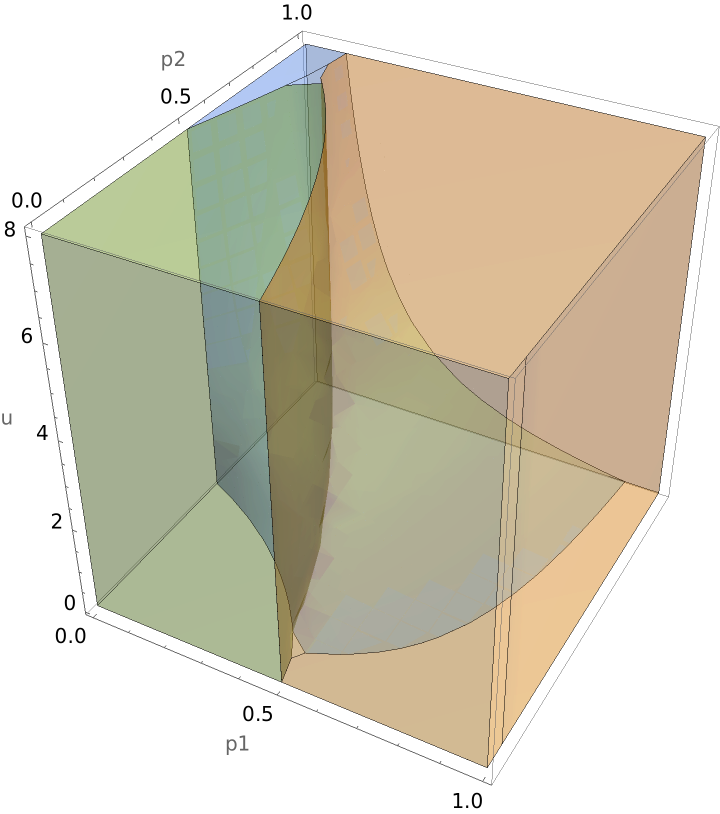Seth J. Chandler

## Version History

• 1.0.0 – 19 October 2020